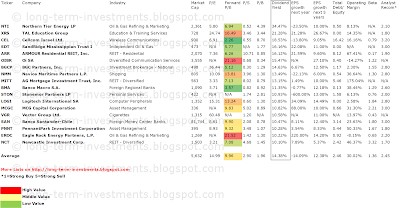## Pages

### 18 Stocks With Very High Yields (+10%) And Earnings Growth

+10% yielding shares with strong earnings per share growth originally published at "long-term-investments.blogspot.com" Some of my friends search regular for stocks with very high yield. I don’t talk about high-yields, stocks with yields between 5-10 percent. I mean stocks with annualized dividend yields of more than 10 percent.

It sounds a bit unserious and I personally would never buy such stocks but I can understand my friends because they have only a low net worth and they try to boost their passive income from dividends.

Let me say one thing: It could not sustainable for any kind of business to pay such a high amount of money to shareholders at a normal valuation. Something must be wrong. But if find an attractive investment, you can boost your dividend income and in ten years your investment paid-off.

Out there are 148 stocks with a yield over 10 percent. I’ve tried to figure out some interesting stocks.

These are my criteria:

- Dividend Yield above 10%
- Earnings per share growth for the next 5Y over 5%
- Market Capitalization over 300 million

Eighteen shares fulfilled these criteria. Seven of them have a current buy or better recommendation.

Here are the 3 most recommended stocks:

Newcastle Investment (NYSE:NCT) has a market capitalization of \$1.50 billion. The company generates revenue of \$292.30 million and has a net income of \$258.87 million. The firm’s earnings before interest, taxes, depreciation and amortization (EBITDA) amounts to \$187.94 million. The EBITDA margin is 64.30 percent (the operating margin is 64.19 percent and the net profit margin 88.56 percent).

Financial Analysis: The total debt represents 90.36 percent of the company’s assets and the total debt in relation to the equity amounts to 1,717.79 percent. Due to the financial situation, a return on equity is not calculable. Twelve trailing months earnings per share reached a value of \$2.71. Last fiscal year, the company paid \$0.40 in the form of dividends to shareholders.

Market Valuation: Here are the price ratios of the company: The P/E ratio is 3.22, the P/S ratio is 5.14 and the P/B ratio is finally 7.02. The dividend yield amounts to 10.10 percent and the beta ratio has a value of 3.32.Long-Term Stock History Chart Of Newcastle Investment (NCT)Long-Term Dividends History of Newcastle Investment (NCT)Long-Term Dividend Yield History of Newcastle Investment (NCT)

PennantPark Investment (NASDAQ:PNNT) has a market capitalization of \$723.29 million. The company generates revenue of \$113.39 million and has a net income of \$63.35 million. The firm’s earnings before interest, taxes, depreciation and amortization (EBITDA) amounts to \$62.74 million. The EBITDA margin is 55.33 percent (the operating margin is 50.60 percent and the net profit margin 55.87 percent).

Financial Analysis: The total debt represents 28.90 percent of the company’s assets and the total debt in relation to the equity amounts to 43.97 percent. Due to the financial situation, a return on equity of 11.19 percent was realized. Twelve trailing months earnings per share reached a value of \$1.22. Last fiscal year, the company paid \$1.12 in the form of dividends to shareholders.

Market Valuation: Here are the price ratios of the company: The P/E ratio is 8.96, the P/S ratio is 6.38 and the P/B ratio is finally 1.07. The dividend yield amounts to 10.28 percent and the beta ratio has a value of 1.67.Long-Term Stock History Chart Of PennantPark Investment (PNNT)Long-Term Dividends History of PennantPark Investment (PNNT)Long-Term Dividend Yield History of PennantPark Investment (PNNT)

TAL Education Group (NYSE:XRS) has a market capitalization of \$728.30 million. The company employs 5,422 people, generates revenue of \$177.52 million and has a net income of \$24.31 million. The firm’s earnings before interest, taxes, depreciation and amortization (EBITDA) amounts to \$26.48 million. The EBITDA margin is 14.92 percent (the operating margin is 11.71 percent and the net profit margin 13.70 percent).

Financial Analysis: The total debt represents 0.00 percent of the company’s assets and the total debt in relation to the equity amounts to 0.00 percent. Due to the financial situation, a return on equity of 14.09 percent was realized. Twelve trailing months earnings per share reached a value of \$0.38. Last fiscal year, the company paid \$0.00 in the form of dividends to shareholders.

Market Valuation: Here are the price ratios of the company: The P/E ratio is 24.47, the P/S ratio is 4.10 and the P/B ratio is finally 3.83. The dividend yield amounts to 21.28 percent and the beta ratio is not calculable.Long-Term Stock History Chart Of TAL Education Group (XRS)Long-Term Dividends History of TAL Education Group (XRS)Long-Term Dividend Yield History of TAL Education Group (XRS)

Take a closer look at the full list. The average P/E ratio amounts to 14.99 and forward P/E ratio is 9.96. The dividend yield has a value of 14.38 percent. Price to book ratio is 1.96 and price to sales ratio 2.90. The operating margin amounts to 30.02 percent and the beta ratio is 1.36. The average stock from the screening results has a debt to equity ratio of 2.46.

Here is the full table with some fundamentals (TTM):18 Stocks With Very High Yields (Click to enlarge)